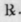The metric system is based upon the meter, which is the standard unit of length of that system, and equal to 39,370,432 inches, or about 10 per cent. longer than the yard.

The metric unit of fiuid measure is the liter - the cube of 1/10 meter, or 1000 cubic centimeters - equal to about 34 fluid ounces.

The metric unit of weight is the Gram, which represents the weight of one cubic-centimeter of water as its maximum density. It is equal to 15 (43,234,874) troy grains.

One cubic-centimeter is equal to 16,231 minims.

In writing prescriptions it is sufficiently accurate and safe to consider i gram as exactly equal to 15 troy grains, and to consider i cubic centimeter as equal to 15 minims.

We accordingly have -

1 gram equal to 15/1 troy grains.

1 troy grain equal to 1/15 gram.

1 cubic centimeter equal to \ fluidrachm.

1 fluidrachm equal to 4/1 cubic centimeter.

Hence -

1. To Convert Troy Grains Into Grams, Or Minims Into Cubic Centimeters---a. Divide by 10, and from the quotient subtract one-third; or, b. Divide by 15; and

2. To Convert Apothecaries' Drachms Into Grams, Or Fluidrachms Into Cubic Centimeters, multiply by 4.

In writing prescriptions, the "gram" (abbreviated "Gm.") and "cubic centimeter" (abbreviated "C.C.," which may be called "fluigram," and written "fGm") only should be used.

The centigram, which is a very convenient unit to refer to in medicine and pharmacy, is used in books and in speaking, but not in writing prescriptions.

All other terms, and units, and prefixes used in the metric system, may be wholly ignored by the physician and the pharmacist. *

The use of a decimal line prevents possible errors.

To write a prescription for fifteen doses of any medicine, write it first for one dose in grains and minims, and then substitute the same number of "grams" and "cube-cents," thus: -Opii..................... gr. j

Camphorae.................. gr. ij

Make one pill. and to get fifteen such doses in metric terms, write -Opii..................... 1 Gm.

Camphorae.................. 2 Gm.

Make fifteen pills.

The gram and the cubic centimeter (fluigram), when referring to liquids, may be considered as equal quantities, except the liquids be very heavy (as in the case of chloroform), or very light (as in the case of ether).

Measures may be discarded and weights exclusively employed, if preferred. All quantities in a prescription would then be expressed in Grams.

* The prefixes are simply numerals, as follows: myria, which means 10,000.

kilo, " " 1,000.

hecto, " " 100.

deka, " " 10.

deci, which means 0.1. centi, '" " 0.01. milli, " " 0.001.

and are quite unnecessary in the writing of prescriptions (if not in all cases), English numerals being more convenient, and at least equally explicit.